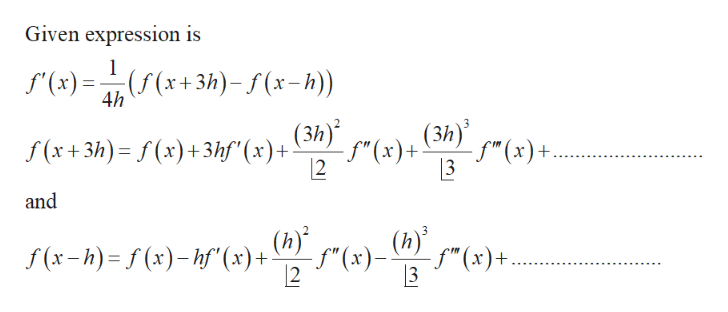# Determine the error term of for the formula:f'(x)  ≈ (1/4h) [f(x+3h) - f(x-h)]

Question
58 views

Determine the error term of for the formula:

f'(x)  ≈ (1/4h) [f(x+3h) - f(x-h)]

check_circle

In order to find the error term of the given expressio...help_outlineImage TranscriptioncloseGiven expression is f"(x)=(f(x+3h)- f(x-h)) (3h) (3h) - f"(x)+ [2 S (x + 3h) = f (x)+3hf"(x)+ - f"(x)+. %3D 3 and (h) f" (x)*- (h) 12 - S"(x)- f (x-h) = f (x)– hf"(x) + fullscreen

### Want to see the full answer?

See Solution

#### Want to see this answer and more?

Solutions are written by subject experts who are available 24/7. Questions are typically answered within 1 hour.*

See Solution
*Response times may vary by subject and question.
Tagged in

### Math